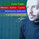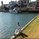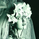14308 lượt xem
A Hull Moving Average strategy. I simply copied the code from mohamed982 and wrapped it in strategy code. All credits go to him not me.
```//@version=2
strategy("HullMA Strategy", overlay=true)

n=input(title="period",type=integer,defval=16)

n2ma=2*wma(close,round(n/2))
nma=wma(close,n)
diff=n2ma-nma
sqn=round(sqrt(n))

n2ma1=2*wma(close,round(n/2))
nma1=wma(close,n)
diff1=n2ma1-nma1
sqn1=round(sqrt(n))

n1=wma(diff,sqn)
n2=wma(diff1,sqn)
c=n1>n2?green:red
ma=plot(n1,color=c)

longCondition = n1>n2
if (longCondition)
strategy.entry("Long", strategy.long)

shortCondition = longCondition != true
if (shortCondition)
strategy.entry("Short", strategy.short)```

## Bình luậnHi, I would ask for 2 features:

1 - The possibility to set alerts.
2 - Enable/Disable the plotting of the line on the chart, leaving visible the signals.

Kind regards

G. Aloe
Phản hồichenchen3529
what settings do I have to use for 2m charting.. please
Phản hồiag580108
I'm actually not at all an active trader :), I'm currently developing an indicator that learn the way a market moves to predict the future tho
Phản hồisirolf2009
you rock bro really, respect from me for all of your strategy
Phản hồibbrox
Calculate a Weighted Moving Average with period n / 2 and multiply it by 2
Calculate a Weighted Moving Average for period n and subtract if from step 1
Calculate a Weighted Moving Average with period sqrt(n) using the data from step 2

Basically, if the line turns green you buy, if the line turns red you sell ;)
Phản hồi